SLOS358C September   2011  – April 2020

PRODUCTION DATA.

1. Features
2. Applications
3. Description
4. Revision History
5. Packaging and Ordering Information
6. Electrical Specifications
7. Device Information
1. 7.1 PIN Configurations
8. Table of Graphs
9. Typical Characteristics: VS = 2.7 V
10. 10Typical Characteristics: VS = 5 V
11. 11Application Information
12. 12Device and Documentation Support
13. 13Mechanical, Packaging, and Orderable Information

• RUN|10
• D|8
• DGK|8
• DGK|8

## 10 Typical Characteristics: VS = 5 V

Test conditions unless otherwise noted: VS+ = 5 V, VS– = 0 V, VOCM = open, VOUT = 2 Vpp, RF = 2 kΩ, RL = 2 kΩ Differential, G = 1 V/V, Single-Ended Input, Differential Output, Input and Output Referenced to mid-supply, TA = 25°C unless otherwise noted.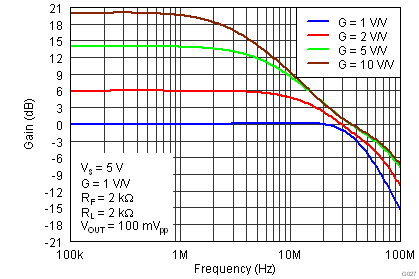Figure 34. Small-Signal Frequency Response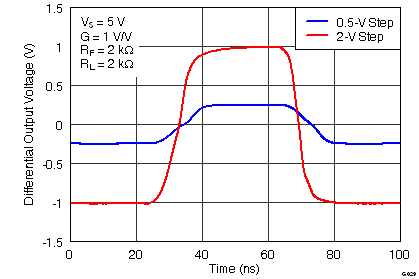Figure 36. Large and Small-Signal Pulse Response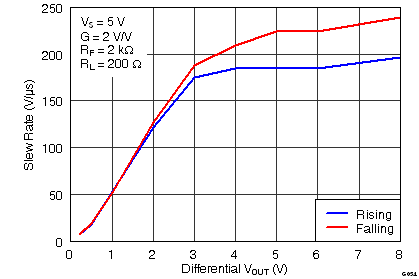Figure 38. Differential Slew Rate vs VOUT StepFigure 40. 10 kHz FFT On Audio AnalyzerFigure 42. Harmonic Distortion vs Output Voltage at 1 MHz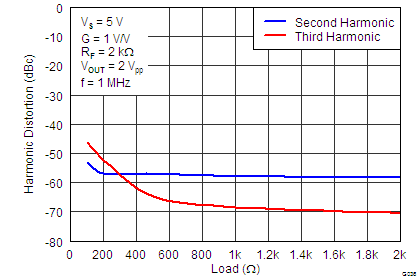Figure 44. Harmonic Distortion vs Load at 1 MHzFigure 46. Two-Tone, 2nd and 3rdOrder Intermodulation Distortion vs FrequencyFigure 48. Single-Ended Output Saturation Voltage vs Load CurrentFigure 50. Frequency Response vs CLOAD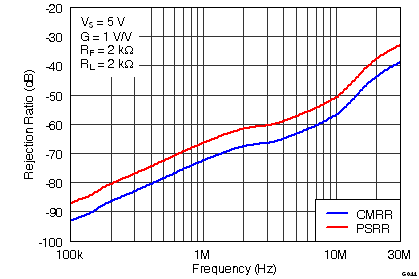Figure 52. Rejection Ratio vs FrequencyFigure 54. Turn-Off Time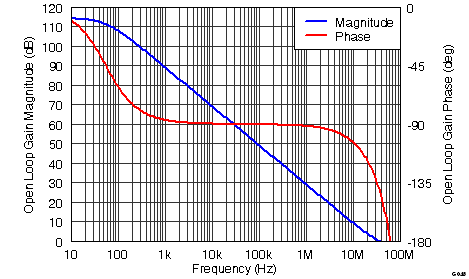Figure 56. Main Amplifier Differential Open-Loop Gain and Phase vs Frequency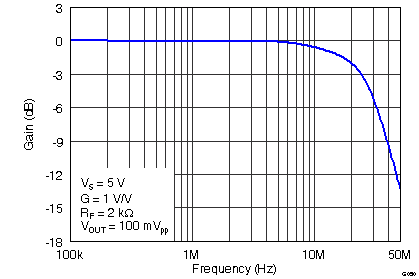Figure 58. VOCM Small-Signal Frequency Response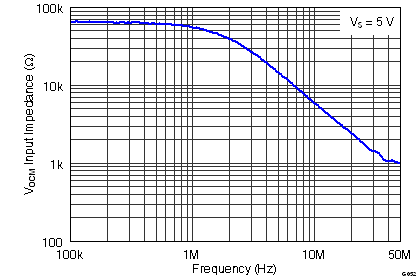Figure 60. VOCM Input Impedance vs Frequency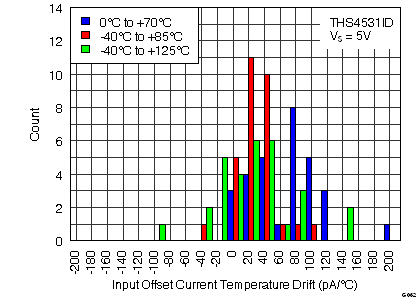Figure 62. Input Offset Current Temperature Drift Histogram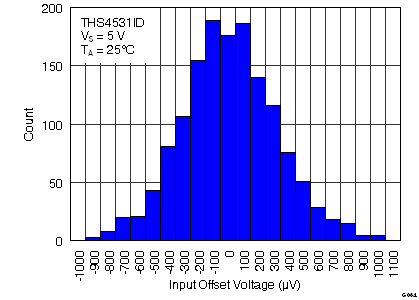Figure 64. Input Offset Voltage HistogramFigure 66. Input Offset Voltage vs Temperature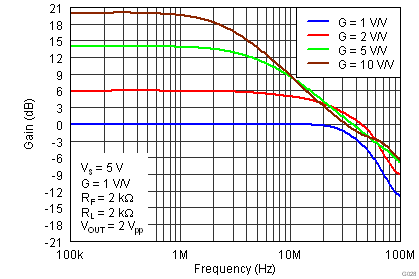Figure 35. Large-Signal Frequency Response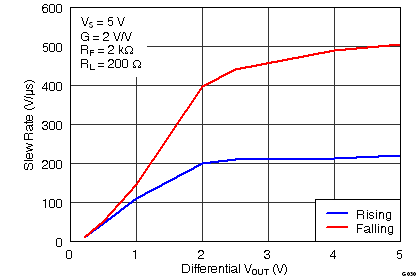Figure 37. Single-Ended Slew Rate vs VOUT Step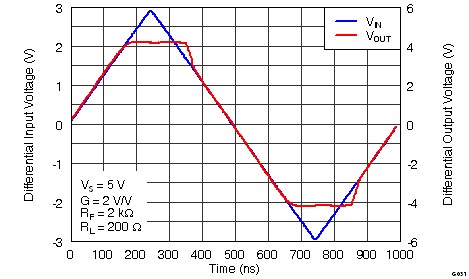Figure 39. Overdrive Recovery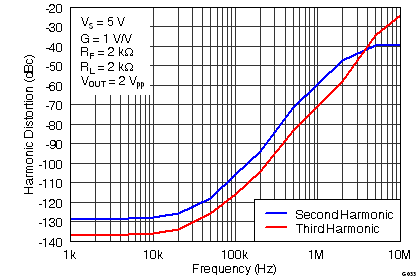Figure 41. Harmonic Distortion vs Frequency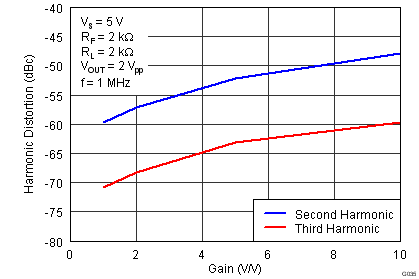Figure 43. Harmonic Distortion vs Gain at 1 MHz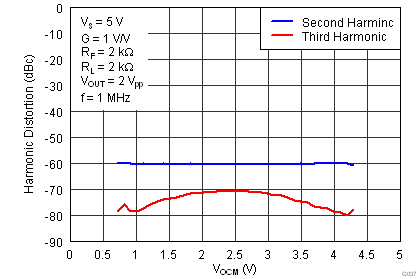Figure 45. Harmonic Distortion vs VOCM at 1 MHzFigure 47. Single-Ended Output Voltage Swing vs Load Resistance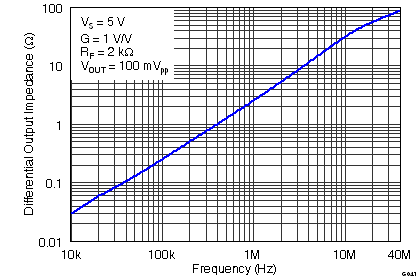Figure 49. Main Amplifier Differential Output Impedance vs Frequency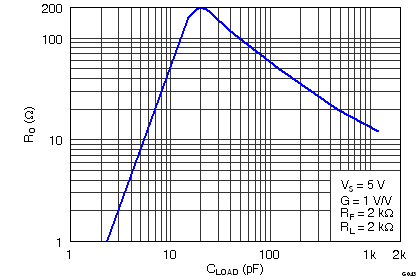Figure 51. RO vs CLOAD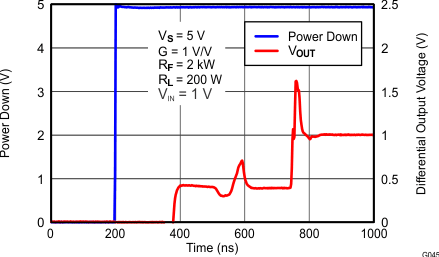Figure 53. Turn-On TimeFigure 55. Input-Referred Voltage Noise and Current Noise Spectral Density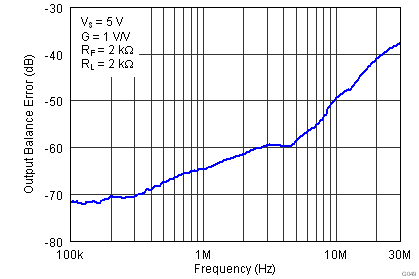Figure 57. Output Balance Error vs FrequencyFigure 59. VOCM Large and Small-Signal Pulse Response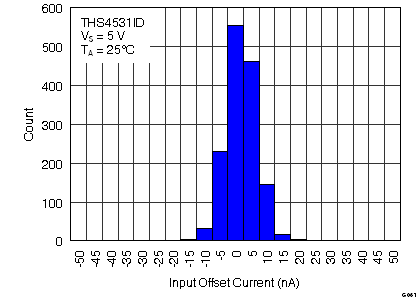Figure 61. Input Offset Current Histogram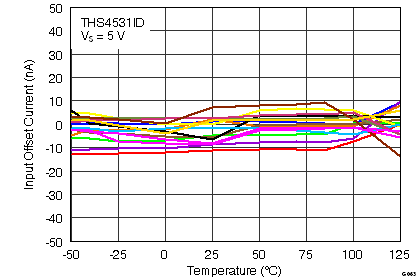Figure 63. Input Offset Current vs Temperature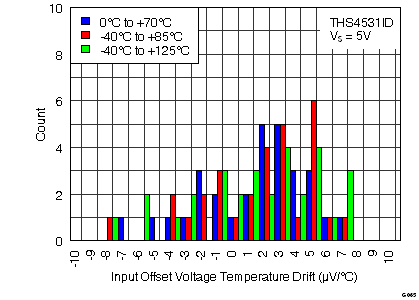Figure 65. Input Offset Voltage Temperature Drift Histogram